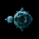706 lượt xem
706
It's a simple indication.
I multiplied the output of bop with volume , make it more smoother.
```study(title="Balance of Power")
length=input(defval=14,minval=1,type=integer)
siglen=input(defval=9,minval=1,type=integer)
vw=input(type=bool,defval=true,title="Volume Weighted")
bp=vw==true ? (close - open) / (high - low)*volume : (close - open) / (high - low)
bop=sma(bp,length)
sig=sma(bop,siglen)
plot(bop)
plot(sig,color=green)
hline(0)```good tool! change the moving average on lines 6 and 7 to WMA and you will see the divergences a little earlier.
Phản hồi